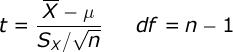# One-Sample T Test Solution

### Example homework problem:

A car company claims that their Super Spiffy Sedan averages 31 mpg. You randomly select 8 Super Spiffies from local car dealerships and test their gas mileage under similar conditions.

You get the following MPG scores:

 MPG: 30 28 32 26 33 25 28 30

Does the actual gas mileage for these cars deviate significantly from 31 (alpha = .05)?

The goal of your analysis is to test for a significant deviation between your sample mean and the proposed population mean.

We will test the mean of these data against the value 31.00 with a one-sample t test of the mean:The numerator of the formula is equal to your sample mean minus the population value you are comparing your sample to (in our case, this is 31). The denominator is equal to the standard error of your sample: the standard deviation of your sample divided by the square root of n (the number of scores in your sample). If you would like help computing the mean and standard deviation, please see the documentation for the explore procedure.

Compute test statistic. Compute the mean and standard deviation of your sample. You will find that the mean is equal to 29.00 and the standard deviation is equal to 2.78. Now, compute your t statistic:

Conduct hypothesis test. The degrees of freedom (df) for your test is equal to n – 1. In this study, df = 8 – 1 = 7. You will be conducting a non-directional (i.e., two-tailed) test with alpha = .05. From a table of the critical values of t, you will see that if the absolute value of your obtained t is greater than 2.365, you would conclude that the average gas mileage of the cars is significantly different from 31.00.

Since your obtained absolute value of t (2.04) is less than the critical value (2.365), you would conclude that the average gas mileage of your sample did not differ significantly from 31.00.